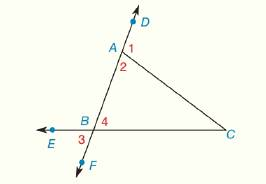Chapter 3.CR, Problem 25CR### Elementary Geometry for College St...

6th Edition
Daniel C. Alexander + 1 other
ISBN: 9781285195698

#### Solutions

Chapter
Section### Elementary Geometry for College St...

6th Edition
Daniel C. Alexander + 1 other
ISBN: 9781285195698
Textbook Problem
1 views

# Given: Δ A B C is isosceles with base A B ¯ A B = y + 7 B C = 3 y + 5 A C = 9 − y Find: Whether Δ A B C is also equilateralExercises 25, 26

To determine

Whether ΔABC is also equilateral.

Explanation

Definition:

The triangle whose all sides and angles are same is called equilateral triangle.

The triangle whose two sides are equal is called isosceles triangle.

Given:

The triangle ΔABC is shown in the figure below,

Figure (1)

From figure (1),

ΔABC is isosceles with base AB¯

AB=y+7BC=3y+5AC=9y

Calculation:

Consider the triangle ΔABC,

From the given data,

The triangle ΔABC is isosceles with base AB¯. Then,

AC=BC (1)

Substitute (9y) for AC and (3y+5) in equation (1)

### Still sussing out bartleby?

Check out a sample textbook solution.

See a sample solution

#### The Solution to Your Study Problems

Bartleby provides explanations to thousands of textbook problems written by our experts, many with advanced degrees!

Get Started

#### Write the sum in sigma notation. 14. 37+48+59+610++2327

Single Variable Calculus: Early Transcendentals, Volume I

#### Using n = 4 and midpoints for xi, then Riemann sum for 17x2 dx is a) 3443 b) 72 c) 168 d) 112

Study Guide for Stewart's Single Variable Calculus: Early Transcendentals, 8th

#### What is the primary factor that determines when it is time to change phases?

Research Methods for the Behavioral Sciences (MindTap Course List)